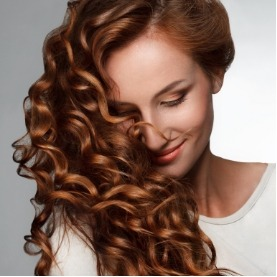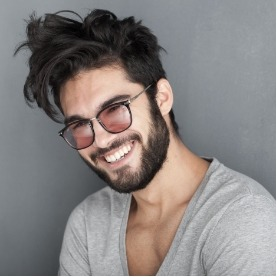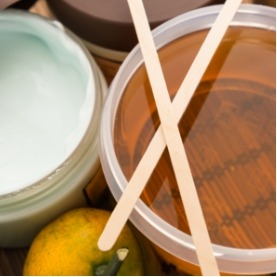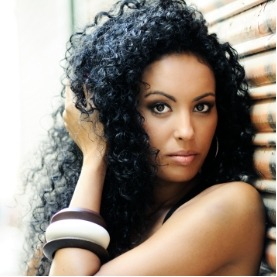•## Color Services

• Single Application (up to 4oz)
\$64 - \$89

Level 1 = \$64 Level 2 = \$69 Level 3 = \$74 Level 4 = \$79 Level 5 = \$84 Level 6 = \$89

• Partial Highlight (11-30 Foils)
\$79 - \$104

Level 1 = \$79 Level 2 = \$84 Level 3 = \$89 Level 4 = \$94 Level 5 = \$99 Level 6 = \$104

• Full Highlight (31+ Foils)
\$94 - \$119

Level 1 = \$94 Level 2 = \$99 Level 3 = \$104 Level 4 = \$109 Level 5 = \$114 Level 6 = \$119

• Balayage
\$144 - \$174

Level 1 = \$144 Level 2 = \$150 Level 3 = \$156 Level 4 = \$162 Level 5 = \$168 Level 6 = \$174

## Haircut Services

• WOMEN'S HAIRCUT/STYLE
\$33-\$54

Level 1 = \$33 Level 2 = \$37 Level 3 = \$41

• WOMEN'S CUT W/ COLOR SERVICE
\$25-\$45

Level 1 = \$25 Level 2 = \$29 Level 3 = \$33

• SHAMPOO BLOW DRY
\$28-\$43

Level 1 = \$28 Level 2 = \$30 Level 3 = \$33

• FLAT IRON / CURLING IRON
\$15

\$15 for each additional 15 minutes. 15-30 minutes is normal. Extra long/thick hair will be 45 minutes.

• SPECIAL OCCASION (Up-Do)
\$58-\$72

Level 1 = \$58 Level 2 = \$60 Level 3 = \$62

• BANG TRIM
\$8-\$13

Level 1 = \$8 Level 2 = \$9 Level 3 = \$10

•• ## MEN’S HAIR

• MEN'S HAIRCUT
\$23-\$44

Level 1 = \$23 Level 2 = \$27 Level 3 = \$31

• CHILDREN'S HAIRCUT
\$23-\$44

We reserve more time for children so that we can perfect an adult haircut/style. Level 1 = \$23 Level 2 = \$27 Level 3 = \$31

• STRAIGHT RAZOR FACE SHAVE
\$23-\$44

Level 1 = \$23 Level 2 = \$27 Level 3 = \$31

• COLOR CAMO (GRAY BLENDING)
\$25

Reduces gray up to 80%, very natural looking. Takes 5-10 minutes at the shampoo bowl. Level 1 = \$25 Level 2 = \$25 Level 3 = \$25

• BEARD TRIM
\$8-\$13

Level 1 = \$8 Level 2 = \$9 Level 3 = \$10

• SHAPE -UP
\$12-\$17

Level 1 = \$12 Level 2 = \$13 Level 3 = \$14

• DESIGN
\$23-\$44

We price our design services per half hour. We charge our Men's haircut price per half hour. (15 minutes would be 50% of your service provider's haircut level price) Level 1 = \$23 Level 2 = \$27 Level 3 = \$31

•• ## WAXING

• BROW SHAPING
\$15

Level 1 = \$15 Level 2 = \$15 Level 3 = \$15

• LIP
\$10

Level 1 = \$10 Level 2 = \$10 Level 3 = \$10

• CHIN
\$10

Level 1 = \$10 Level 2 = \$10 Level 3 = \$10

• FULL FACE
\$28

Level 1 = \$28 Level 2 = \$28 Level 3 = \$28

•• ## TREATMENTS

• HEAT CURE TREATMENT
\$35

This is a specialized service that uses it's own heating tool in order to apply. Level 1 = \$35 Level 2 = \$35 Level 3 = \$35

• PH BONDER TREATMENT
\$20

Level 1 = \$20 Level 2 = \$20 Level 3 = \$20

• CHEMISTRY SYSTEM TREATMENT
\$15

Level 1 = \$15 Level 2 = \$15 Level 3 = \$15

• PRE-ART TREATMENT
\$15

Level 1 = \$15 Level 2 = \$15 Level 3 = \$15

• CAT TREATMENT
\$15

Intense damage treatment. Level 1 = \$15 Level 2 = \$15 Level 3 = \$15

\$6

Level 1 = \$6 Level 2 = \$6 Level 3 = \$6

\$6

Level 1 = \$6 Level 2 = \$6 Level 3 = \$6